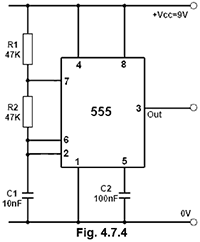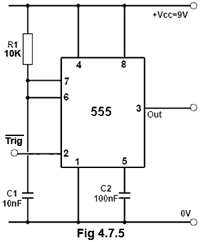# Square Wave Oscillators Quiz

Try this quiz, based on the information you can find in Oscillators Module 4. Submit your answers and see how many you get right. If you get any answers wrong. Just follow the hints to find the right answer and learn about Oscillators as you go.

### 1.

What is the duty cycle of a square wave having a mark to space ratio of 2:1

### 2.

What is the p.r.f. of the wave shown in Fig. 4.7.1?### 3.

Refer to Fig. 4.7.2. What is the purpose of D1 and D2?### 4.

In the op-amp astable circuit shown in Fig. 4.7.3, which of the following op-amp characteristics limits the highest useful frequency of the circuit?### 5.

Refer to Fig. 4.7.3. What is its approximate frequency of oscillation?

### 6.

Refer to Fig. 4.7.4. Which of the following would be the approximate output frequency?### 7.

Refer to Fig. 4.7.4. What will be the mark to space ratio of the output waveform on pin 3 of the 555 timer?

### 8.

Refer to Fig. 4.7.4. What voltage reading would be expected if a DC voltmeter is connected to Pin 5 of the 555 timer?

### 9.

Refer to Fig 4.7.5. If a 2µs trigger pulse is applied to pin 2, what will be the approximate duration of the output pulse at pin 3?### 10.

Refer to Fig 4.7.5. To obtain a continuous output waveform with a 50% duty cycle, what would be the required p.r.f. of the repeated trigger pulses applied to pin 2?

Top of Page